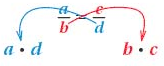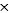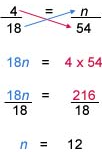Solve Proportions

Solve Proportions

True Proportions

• A proportion is an expression of the equality of two ratios or rates.
• A proportion is true if the fractions are equal when written in lowest terms.

Example

Any units expressed in a proportion must be consistent (when units are used, the units of the numerators are the same, and the units of the denominators are the same.) Also, in any true proportion, the "cross products" are equal.

The cross products ad and bc are obtained from the proportionWe know that 312 = 49 from the proportion

Solving Proportions

Sometimes one of the numbers in a proportion is unknown. In this case, it is necessary to solve the proportion.

To solve the proportion, find a number to replace the unknown so that the proportion is true. You can use cross-products to solve a proportion.

Example

Find n on the following proportion

Solution